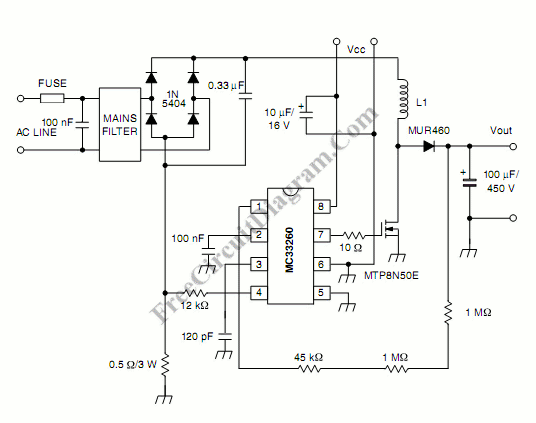# Active Power Factor Correction CircuitActive power factor correction stabilize the electrical demand of a device to give the best power factor characteristic of many types of loads.  To meet power factor regulation, a low cost solution should be designed. In many application, the need of high DC voltage is usually implemented by a direct rectification of the AC line followed by bulk capacitor filtering. This capacitor filtering introduce current spike that distort the power line sine waveform, and this introduce a poor power factor, resulting in an apparent input power that is much higher than the real power. This can be solved by inserting a pre-regulation between the rectifier and the bulk capacitor. We call this pre-regulator circuit as power factor correction circuit.  Here is the schematic diagram of the circuit:This power factor controller is a low–cost system solution for boost mode follower. that meets IEC1000–3–2 standard. This power factor correction circuit includes an inrush current detection, protection against overcurrent, overvoltage and undervoltage. Follower boost mode for system cost reduction – smaller inductor and MOSFET can be used. [Circuit schematic source: ON Semiconductor Application Notes]